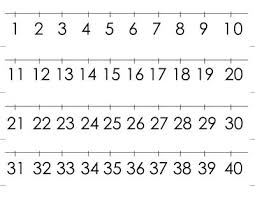# Percentage 67364

Create all four-digit numbers in which the digits 0, 2, 5, and 9 do not repeat.
A) How many such numbers are there? You solve using a tree diagram.
B) What percentage of them are even?

a =  18
p =  55.5556 %

### Step-by-step explanation:Did you find an error or inaccuracy? Feel free to write us. Thank you!

Tips for related online calculators
Need help calculating sum, simplifying, or multiplying fractions? Try our fraction calculator.